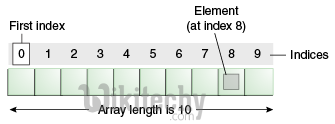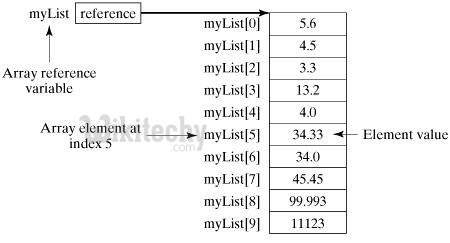# java tutorial - Java Arrays - tutorial java - java programming - learn java - java basics- java for beginners

Arrays in Java are a data structure that stores ordered collections of fixed-sized elements of the desired type.

• In Java, an array is used to store a collection of data, but it is often useful to think of an array as a collection of variables of the same type.
• Instead of declaring individual variables, such as number0, number1, ..., and number99, you declare one array variable, for example, numbers and use numbers , numbers , ..., and numbers  for mapping of individual variables.
• This article will introduce you to how to declare an array of variables in Java, create and manipulate an array using indexed variables.Learn java - java tutorial - java array - java examples - java programs

## Declaring an array

To use an array in a program, you need to declare a variable to reference the array, and you must specify the type of the array that can reference the variable. The syntax for declaring an array variable is:

Note: The style dataType[] arrayRefVar is preferred.

## Sample Code

The following code snippets are examples of using this syntax:

## Creating an array

In Java, you can create an array using the new operator using the following syntax:

The above declaration does two things:

• Creates an array using the new dataType [arraySize];
• A reference to the new created array is assigned to the variable arrayRefVar.

Declaring a variable, creating and assigning an array reference variable can be shown below:

Alternatively, arrays in Java can be created as follows:

• Array elements are available to the index. Indices are counted from 0; that is, they start from 0 to arrayRefVar.length-1.

## Sample Code

• The following statement declares an array of variables myList, creates an array of 10 elements of type double and assigns the link myList:
• The image displays the array myList. Here myList has ten double values and indices from 0 to 9.Learn java - java tutorial - java array - java examples - java programs

## Working with arrays

When working with array elements, the for loop or foreach loop is often used because all elements have the same type and known size.

## Sample Code

A complete example showing how to create, initialize, and process an array:

## Foreach cycle

JDK 1.5 introduced a new for loop , known as a foreach loop or an extended for loop , which allows you to sequentially traverse an entire array without using a variable index.

## Sample Code

The following code displays all the elements in the myList array:

## Passing an array to a method

• Just as you can pass primitive type values to methods, you can also pass arrays to methods. For example, the following method displays the elements in an int array −
• You can invoke it by passing an array. For example, printArray method to display 3, 1, 2, 6, 4, and 2 :

## Returning an Array from a Method

• A method may also return an array. For example, the following method returns an array that is the reversal of another array :

## Methods for an array

The java.util.Array class takes several static methods for sorting and searching arrays, comparing arrays, and filling array elements.

Sr.No. Method & Description
1

public static int binarySearch(Object[] a, Object key)

Using the binary search algorithm it can searches the specified array Object ( Byte, Int , double, etc.).if it is contained in the list it returns index of the search key,otherwise, it returns ( – (insertion point + 1)).

2

public static boolean equals(long[] a, long[] a2)

Two arrays are considered equivalent if both arrays contain the same number of elements, and all corresponding pairs of elements in the two arrays are same this returns true.

3

public static void fill(int[] a, int val)

It Assigns the specified array of value to Int.

4

public static void sort(Object[] a)

Sorts the specified array of objects into an ascending order,the same method could be used by all other primitive data types ( Byte, short, Int, etc.)

## Example 2: the maximum element of an array

• Simple ways to find the maximum number in an array in Java. First, use the Math.max () method .